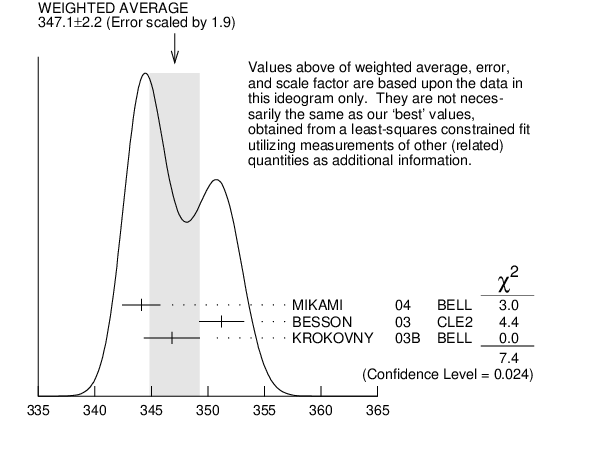# ${\boldsymbol m}_{{{\boldsymbol D}_{{s1}}{(2460)}^{\pm}}}–{\boldsymbol m}_{{{\boldsymbol D}_{{s}}^{*\pm}}}$ INSPIRE search

The fit includes ${{\mathit D}^{\pm}}$, ${{\mathit D}^{0}}$, ${{\mathit D}_{{s}}^{\pm}}$, ${{\mathit D}^{*\pm}}$, ${{\mathit D}^{*0}}$, ${{\mathit D}_{{s}}^{*\pm}}$, ${{\mathit D}_{{1}}{(2420)}^{0}}$, ${{\mathit D}_{{2}}^{*}{(2460)}^{0}}$, and ${{\mathit D}_{{s1}}{(2536)}^{\pm}}$ mass and mass difference measurements.
VALUE (MeV) EVTS DOCUMENT ID TECN  COMMENT
$\bf{ 347.3 \pm0.7}$ OUR FIT  Error includes scale factor of 1.2.
$\bf{ 347.1 \pm2.2}$ OUR AVERAGE  Error includes scale factor of 1.9.
$344.1$ $\pm1.3$ $\pm1.1$ 126
 2004
BELL 10.6 ${{\mathit e}^{+}}{{\mathit e}^{-}}$
$351.2$ $\pm1.7$ $\pm1.0$ 41
 2003
CLE2 10.6 ${{\mathit e}^{+}}{{\mathit e}^{-}}$
$346.8$ $\pm1.6$ $\pm1.9$ 57 1
 2003 B
BELL 10.6 ${{\mathit e}^{+}}{{\mathit e}^{-}}$
1  Recalculated by us using ${\mathit m}_{{{\mathit D}_{{s}}^{*+}}}$ = $2112.4$ $\pm0.7$ MeV.${\mathit m}_{{{\mathit D}_{{s1}}{(2460)}^{\pm}}}–{\mathit m}_{{{\mathit D}_{{s}}^{*\pm}}}$ (MeV)
References:
 MIKAMI 2004
PRL 92 012002 Measurements of the ${{\mathit D}_{{sJ}}}$ Resonance Properties
 BESSON 2003
PR D68 032002 Observation of a Narrow Resonance of Mass 2.46 GeV/$\mathit c{}^{2}$ Decaying to ${{\mathit D}_{{s}}^{*+}}{{\mathit \pi}^{0}}$ and confirmation of the ${{\mathit D}_{{sJ}}^{*}{(2317)}}$ State
 KROKOVNY 2003B
PRL 91 262002 Observation of the ${{\mathit D}_{{sJ}}{(2317)}}$ and ${{\mathit D}_{{sJ}}{(2457)}}$ in ${{\mathit B}}$ Decays